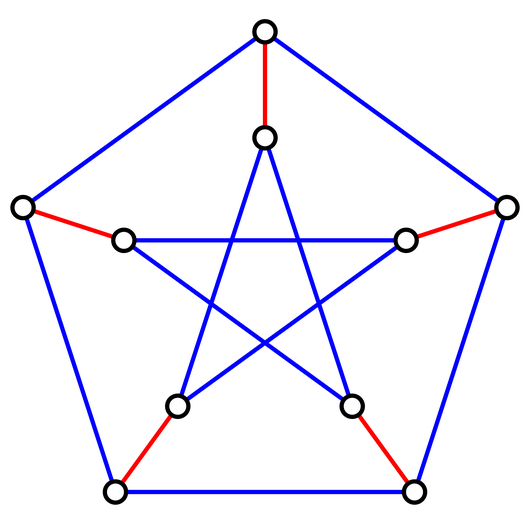# Glossary

## Perfect matching

A perfect matching in a graph is a matching that includes every node in the graph. For the matching to include every node, the underlying graph must contain an even total number of nodes.

For example, the five highlighted red edges in the graph below form a perfect matching on the graph's 10 nodes.It is worth noting that a graph simply containing an even number of nodes does not guarantee the existence of a perfect matching in the graph.

Let $p_n$ denote the total number of perfect matchings in the complete graph $K_n$. To count $p_n$, select any node $x$, and note that we may connect $x$ to one of $2n-1$ other nodes, which leaves us with $2n-2$ nodes to match however we like. This reasoning yields the recurrence relation $p_n = (2n-1) \cdot p_{n-1}$, which when combined with the fact that $p_1 = 1$ provides the closed formula $p_n = (2n-1) \cdot (2n-3) \cdots (5)(3)$.